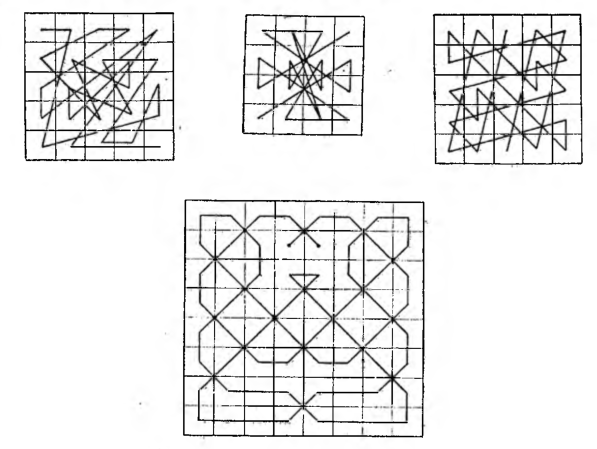# Geometric diagrams of magic squares

Geometric diagrams of magic squares

The structure of each magic square can be illustrated with the help of diagrams showing the sequence of numbers in the fields, and they often bind these lines into very interesting figures. In the drawings we give diagrams of a 4X4 square, two different methods of juxtaposed squares 5 X 5 and the unusually symmetrical 8X8 square diagram. It is worth considering the construction of the last square in detail, substituting the numbers from 1 into 64 in any direction from the exit points to the right or left.